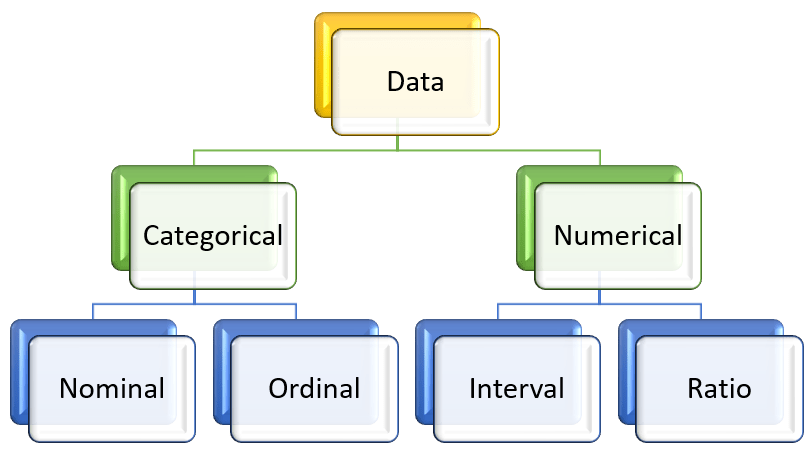# Types of Data

Data Types are an important concept of statistics, which needs to be understood, to correctly apply statistical measurements to your data and therefore to correctly conclude certain assumptions about it. This blog post will introduce you to the different data types you need to know, to do proper exploratory data analysis (EDA), which is one of the most underestimated parts of a machine learning project.Categorical Data

Categorical data represents characteristics. Therefore it can represent things like a person’s gender, language etc. Categorical data can also take on numerical values (Example: 1 for female and 0 for male). Note that those numbers don’t have mathematical meaning.

Nominal Data

Nominal values represent discrete units and are used to label variables, that have no quantitative value. Just think of them as “labels”. Note that nominal data that has no order. Therefore, if you would change the order of its values, the meaning would not change.

The nominal data are examined using the grouping method. In this method, the data are grouped into categories, and then the frequency or the percentage of the data can be calculated. These data are visually represented using the pie charts.

Ordinal Data

Ordinal values represent discrete and ordered units. It is therefore nearly the same as nominal data, except that it’s ordering matters.

The ordinal data is commonly represented using a bar chart. These data are investigated and interpreted through many visualization tools. The information may be expressed using tables in which each row in the table shows the distinct category.

Numerical Data

1. Discrete Data

We speak of discrete data if its values are distinct and separate. In other words: We speak of discrete data if the data can only take on certain values. This type of data can’t be measured but it can be counted. It basically represents information that can be categorized into a classification. An example is the number of heads in 100 coin flips.

You can check by asking the following two questions whether you are dealing with discrete data or not: Can you count it and can it be divided up into smaller and smaller parts?

1. Continuous Data

Continuous Data represents measurements and therefore their values can’t be counted but they can be measured. An example would be the height of a person, which you can describe by using intervals on the real number line.

Interval Data

Interval values represent ordered units that have the same difference. Therefore we speak of interval data when we have a variable that contains numeric values that are ordered and where we know the exact differences between the values.

The problem with interval values data is that they don’t have a „true zero“. That means in regards to our example, that there is no such thing as no temperature. With interval data, we can add and subtract, but we cannot multiply, divide or calculate ratios. Because there is no true zero, a lot of descriptive and inferential statistics can’t be applied.

Ratio Data

Ratio values are also ordered units that have the same difference. Ratio values are the same as interval values, with the difference that they do have an absolute zero. Good examples are height, weight, length etc.

error: Content is protected !!